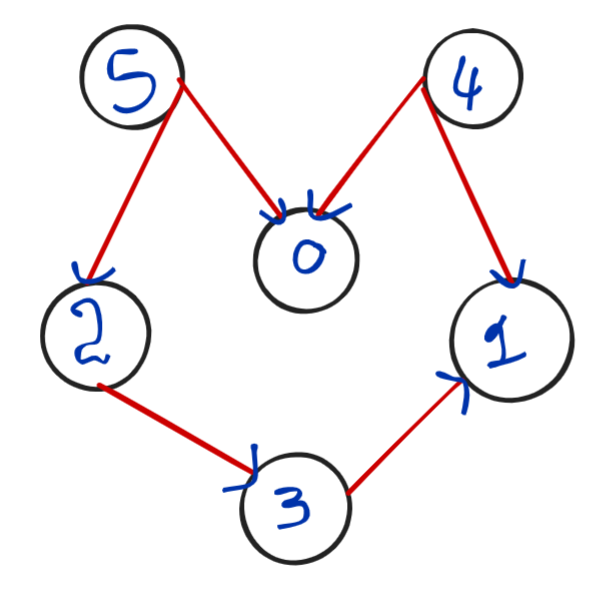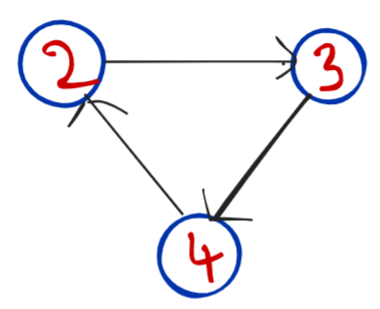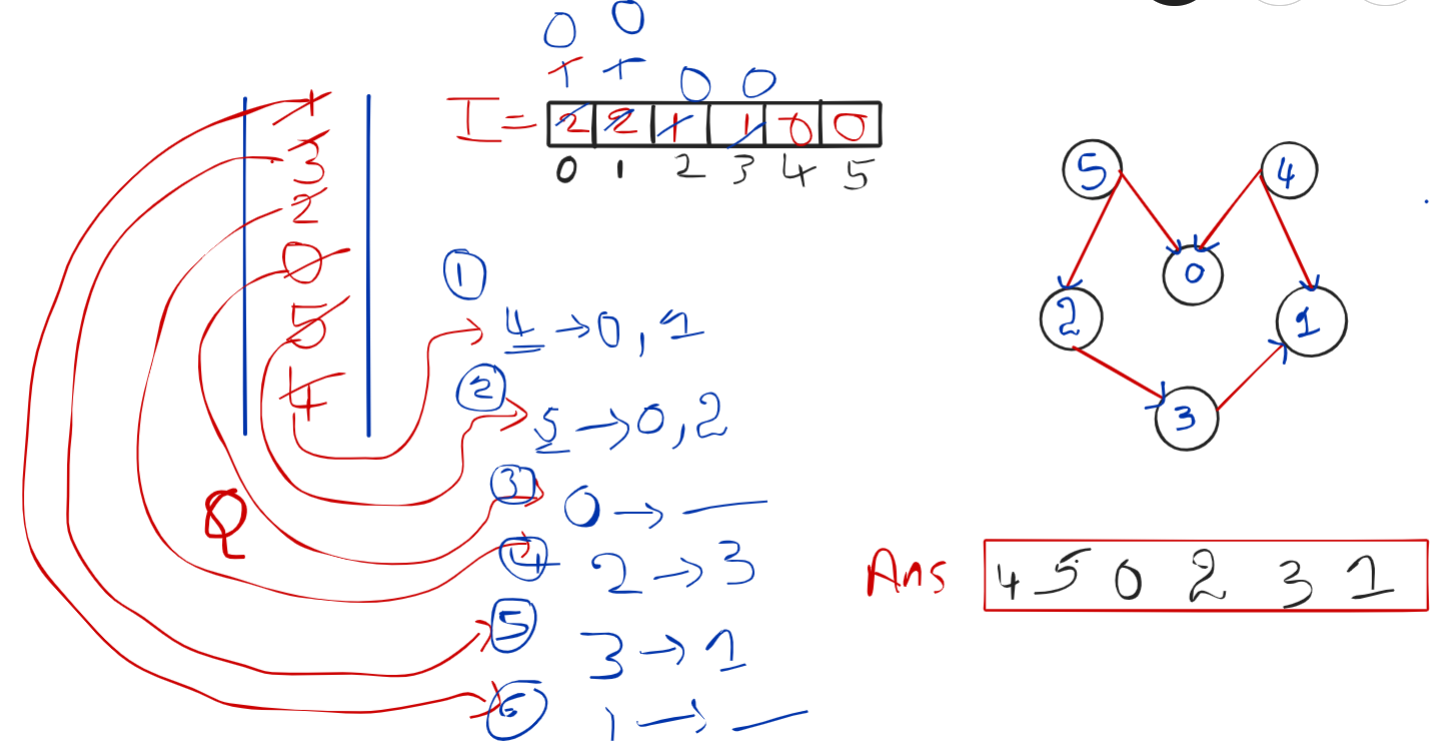# Topological Sort (BFS)

Problem statement: Given a graph, find the topological order for the given graph.

Topological sort: The linear ordering of nodes/vertices such that if there exists an edge between 2 nodes u,v then ‘u’ appears before ‘v’.

Example:

```Example:

Input:Output: 4 5 2 0 3 1

Explanation: 4 is appearing before its neighbours (1,0)
5 is appearing before its neighbours (0,2)
2 is appearing before its neighbours (3)
3 is appearing before its neighbours (1)
```

Note: This can be possible only for DAG ( Directed acyclic graph) because in an undirected graph we can’t decide which node will come first because there will be no direction, and if there is a cycle topological order will not be possible (See below figure to understand why it is not possible for graphs containing cycle).2 ->3 ->4 ->2 here if you can observe

2 is appearing before 3

3 is appearing before 4

4 is appearing before 2

But 2 has already appeared before 4, So topological ordering will not be possible

Intuition

The question states that if there is an edge between u and v then u should appear before v, Which means we have to start this question from a node that doesn’t have any previous edges. But how to find that node that has no edge before if? Here, we use the concept of in-degree (Number of edges pointing toward a node). We find an in-degree which has indegree=0 and we start from this. We use the Indegree concept to find topological sorting. Let’s see how.

Approach:

1. In order to maintain the In-degree of each and every node, we take an array of size v( where v is the number of nodes).
2. Find in-degree of all nodes and fill them in an array
3. Now take Queue data structure and add nodes that have in-degree is 0 (as we discussed in the intuition), and simply apply bfs on queue with some condition.
4. Take the top/peek node from Queue ( let the popped node be x), add this x to our answer. (If you can observe clearly the above step is nothing but Normal BFS traversal).

We’ll apply some conditions to the BFS:
1. Now take neighbour nodes of popped nodes and reduce their in-degree by 1.
2. Check if any of the popped element nodes in degree becomes 0, after reducing in-degree by 1 if it happens then add this neighbour element to the queue for which the in-degree became zero.
5. Repeat step 4 till the queue becomes empty.

We’ll apply some conditions to the BFS:

a) Now take neighbour nodes of popped nodes and reduce their indegree by 1.

b) Check if any of popped element nodes in degree become 0, after reducing in-degree by 1, if it happens then add this neighbour element which in-degree became 0 to the queue.

Let’s see how it works pictorially:Code:

## C++ Code

``````#include<bits/stdc++.h>
using namespace std;

class Solution {
public:
vector<int> topo(int N, vector<int> adj[]) {
queue<int> q;
vector<int> indegree(N, 0);
for(int i = 0;i<N;i++) {
for(auto it: adj[i]) {
indegree[it]++;
}
}

for(int i = 0;i<N;i++) {
if(indegree[i] == 0) {
q.push(i);
}
}
vector<int> topo;
while(!q.empty()) {
int node = q.front();
q.pop();
topo.push_back(node);
for(auto it : adj[node]) {
indegree[it]--;
if(indegree[it] == 0) {
q.push(it);
}
}
}
}
};

int main()
{

Solution obj;
for(auto it:v)
cout<<it<<" ";

return 0;
}``````

Output:

4 5 2 0 3 1

Time Complexity: O(N+E)

Space complexity: O(N)+O(N)

## Java Code

``````import java.util.*;
import java.io.*;
import java.lang.*;

class Solution
{
public boolean isCyclic(int N, ArrayList<ArrayList<Integer>> adj) {
int topo[] = new int[N];
int indegree[] = new int[N];

//finding indegree
for(int i = 0;i<N;i++) {
for(Integer it: adj.get(i)) {
indegree[it]++;
}
}

Queue<Integer> q = new LinkedList<Integer>();
for(int i = 0;i<N;i++) {
//adding nodes to queue with indegree = 0
if(indegree[i] == 0) {
}
}

int cnt = 0;
int ind=0;

while(!q.isEmpty()) {
Integer node = q.poll();
topo[ind++] = node;
cnt++;
//getting neighbour nodes of popped node and decreasing  their
indegree by1
for(Integer it: adj.get(node)) {
indegree[it]--;
if(indegree[it] == 0) {
}
}
}
//printing topological ordering of nodes
for (int i=0;i< topo.length;i++){
System.out.print(topo[i]+" ");
}
if(cnt == N) return false;
return true;
}
}
public class TopoLogicalSortBFS {
public static void main(String args[]) {

ArrayList < ArrayList < Integer >> adj = new ArrayList < > ();

// adding new arraylists to 'adj' to add neighbour nodes
for (int i = 0; i < 6; i++) {
}

}
}
``````

Output:

4 5 2 0 3 1

Time Complexity: O(N+E)

Space complexity: O(N)+O(N)

Special thanks to Sai bargav Nellepalli for contributing to this article on takeUforward. If you also wish to share your knowledge with the takeUforward fam, please check out this article